Courses

# Test: Character Puzzles- 2

## 25 Questions MCQ Test UPSC CSAT: Logical Reasoning (LR) & Data Interpretation (DI) | Test: Character Puzzles- 2

Description
This mock test of Test: Character Puzzles- 2 for UPSC helps you for every UPSC entrance exam. This contains 25 Multiple Choice Questions for UPSC Test: Character Puzzles- 2 (mcq) to study with solutions a complete question bank. The solved questions answers in this Test: Character Puzzles- 2 quiz give you a good mix of easy questions and tough questions. UPSC students definitely take this Test: Character Puzzles- 2 exercise for a better result in the exam. You can find other Test: Character Puzzles- 2 extra questions, long questions & short questions for UPSC on EduRev as well by searching above.
QUESTION: 1

### Which one will replace the question mark ?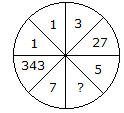Solution:

All numbers are cubed,

(7)3 = 343

(1)3 = 1

(3)3 = 27

Similarly, (5)3 = 125.

QUESTION: 2

### Which one will replace the question mark ?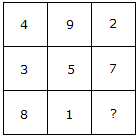Solution:

(4 + 9 + 2) = (3 + 5 + 7) = (8 + 1 + 6)

Total in each case = 15.

QUESTION: 3

### Which one will replace the question mark ?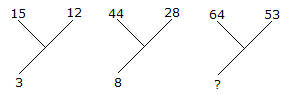Solution:

(15 + 12)/9 = 3

and (44 + 28)/9 = 8

Therefore, (64 + 53)/9 = 13.

QUESTION: 4

Which one will replace the question mark ?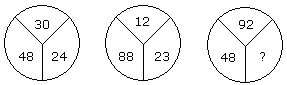Solution:

(30 - 24) x 8 = 48

and (23 - 12) x 8 = 88

Therefore, (92 - 86) x 8 = 48.

QUESTION: 5

Which one will replace the question mark ?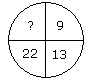Solution:

9 + (2)2 = 13

13 + (3)2 = 22

22 + (4)2 = 38.

QUESTION: 6

Which one will replace the question mark ?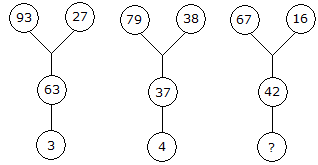Solution:

93 - (27 + 3) = 63

79 - (38 + 4) = 37

Therefore, 67 - (16 + X) = 42

X = 9

QUESTION: 7

Which one will replace the question mark ?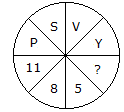Solution:

Putting the position of the letters in reverse order

P = 11, S = 8, V = 5 and Y = 2.

QUESTION: 8

Which one will replace the question mark ?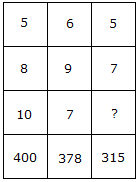Solution:

5 x 8 x 10 = 400

and 6 x 9 x 7 = 378

Therefore 5 x 7 x ? = 315

? = 9.

QUESTION: 9

Which one will replace the question mark ?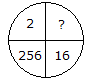Solution:

(16)2 = 256

Therefore, (2)2 = 4.

QUESTION: 10

Which one will replace the question mark ?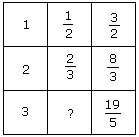Solution:

From I row, 1 + (1/2) = 3/2

From II row, 2 + (2/3) = 8/3

From III row, 3 + ? = 19/5

? = (19/5) - 3

? = (4/5).

QUESTION: 11

Which one will replace the question mark ?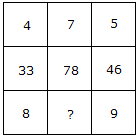Solution:

(4 x 8) + 1 = 33

(5 x 9) + 1 = 46

Similarly, (7 x 11) + 1 = 78.

QUESTION: 12

Which one will replace the question mark ?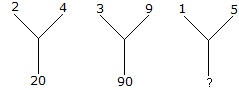Solution:

(2)2 + (4)2 = 20

(3)2 + (9)2 = 90

Therefore, (1)2 + (5)2 = 26.

QUESTION: 13

Which one will replace the question mark ?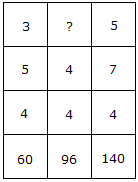Solution:

3 x 5 x 4 = 60

and 5 x 7 x 4 = 140

Therefore, 4 x 4 x ? = 96

? = (96/16) = 6.

QUESTION: 14

Which one will replace the question mark ?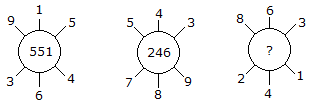Solution:

(915 - 364) = 551

(789 - 543) = 246

(863 - 241) = 622.

QUESTION: 15

Which one will replace the question mark ?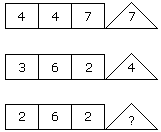Solution:

(4 x 7) % 4 = 7

and (6 x 2) % 3 = 4

Therefore, (6 x 2) % 2 = 6.

QUESTION: 16

Which one will replace the question mark ?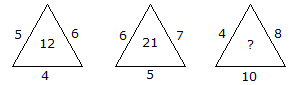Solution:

(5 x 6 x 4)/10 = 12

and (6 x 7 x 5)/10 = 21

Therefore (4 x 8 x 10)/10 = 32.

QUESTION: 17

Which one will replace the question mark ?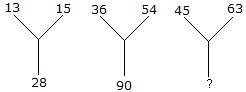Solution:

13 + 15 = 28

36 + 54 = 90

Therefore, 45 + 63 = 108.

QUESTION: 18

Which one will replace the question mark ?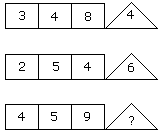Solution:

(3 x 4 - 8) = 4

(2 x 5 - 4) = 6

(4 x 5 - 9) = 11.

QUESTION: 19

Which one will replace the question mark ?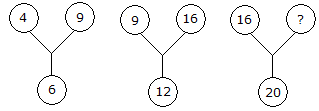Solution: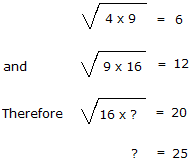QUESTION: 20

Which one will replace the question mark ?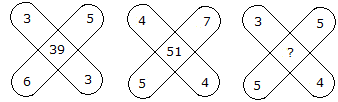Solution:

(3 x 3) + (5 x 6) = 39

and (4 x 4) + (5 x 7) = 51

Therefore, (3 x 4) + (5 x 5) = 37.

QUESTION: 21

Which one will replace the question mark ?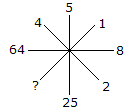Solution:

(2)2 = 4

(8)2 = 64

(5)2 = 25

(1)2 = 1.

QUESTION: 22

Which one will replace the question mark ?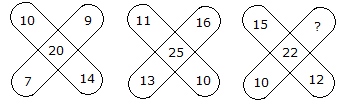Solution:

(10 + 9 + 14 + 7)/2 = 20

and (11 + 16 + 10 + 13)/2 = 25

Therefore, (15 + ? + 12 + 10)/2 = 22

Hence 37 + ? = 44

? = 44 - 37

? = 7.

QUESTION: 23

Which one will replace the question mark ?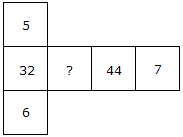Solution:

(5 x 6) + 2 = 32

(7 x 6) + 2 = 44

(7 x 5) + 2 = 37.

QUESTION: 24

Which one will replace the question mark ?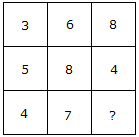Solution:

(5 + 3)/2 = 4

and (6 + 8)/2 = 7

Therefore (8 + 4)/2 = 6.

QUESTION: 25

Which one will replace the question mark ?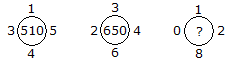Solution:

(1)2 + (5)2 + (4)2 + (3)2 = 51 x 10 = 510

and (3)2 + (4)2 + (6)2 + (2)2 = 65 x 10 = 650

Similarly (0)2 + (1)2 + (2)2 + (8)2 = 69 x 10 = 690.